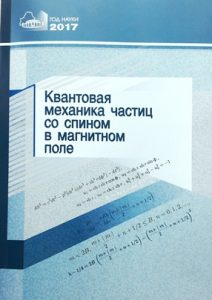# BOOKS

## 2017

QUANTUM MECHANICS OF PARTICLES WITH SPIN IN A MAGNETIC FIELD

National Academy of Sciences of Belarus, B.I. Stepanov Institute of Physics
The authors: Е.М. Овсиюк, О.В. Веко, Я.А. Войнова, В.В. Кисель, В.М. Редьков.ISBN: 978-985-08-2132-4
The year of publishing: 2017
Publisher: Беларуская навука

The monograph presents a tetrad method of generalization of equations for particles of different spins, taking into account the non-Euclidean space-time geometry. In spaces of constant curvature of Lobachevsky and Riemann, exact solutions of the Schrödinger and Dirac equations in an external magnetic field were found. On the basis of the Duffin – Kemmer – Pöthe matrix formalism in Minkowski space, exact solutions of the relativistic equation for a particle with spin 1 in an external magnetic field are found, and this problem is also analyzed in the nonrelativistic approximation.

In the presence of a magnetic field in Minkowski space, exact solutions are constructed for generalized equations for scalar and vector particles that carry, in addition to electric charge, an additional electromagnetic characteristic, polarizability. In the Minkowski space, the Dirac equation takes into account additional interaction through the anomalous magnetic moment of the particle, and exact solutions of this generalized equation are constructed in the presence of homogeneous magnetic and electric fields. The behavior of particles with spins of 0, 1/2, and 1 in a magnetic field is investigated under the restriction to 2-dimensional Lobachevsky and Riemann planes. The quantum-mechanical behavior of a Cox particle, a scalar particle with an additional internal structure, has been studied in external electric and magnetic fields. The cases of all three geometries of space are considered: Euclid, Lobachevsky and Riemann.

It is addressed to researchers, university lecturers, as well as graduate students and students,
specializing in theoretical physics.